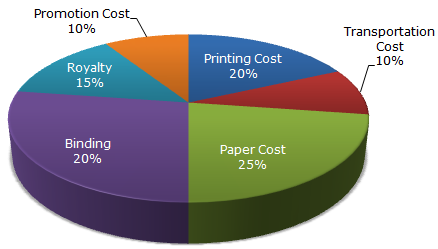# Data Interpretation - Pie Charts

## Why Data Interpretation Pie Charts?

In this section you can learn and practice Data Interpretation Questions based on "Pie Charts" and improve your skills in order to face the interview, competitive examination and various entrance test (CAT, GATE, GRE, MAT, Bank Exam, Railway Exam etc.) with full confidence.

## Where can I get Data Interpretation Pie Charts questions and answers with explanation?

IndiaBIX provides you lots of fully solved Data Interpretation (Pie Charts) questions and answers with Explanation. Solved examples with detailed answer description, explanation are given and it would be easy to understand. All students, freshers can download Data Interpretation Pie Charts quiz questions with answers as PDF files and eBooks.

## Where can I get Data Interpretation Pie Charts Interview Questions and Answers (objective type, multiple choice)?

Here you can find objective type Data Interpretation Pie Charts questions and answers for interview and entrance examination. Multiple choice and true or false type questions are also provided.

## How to solve Data Interpretation Pie Charts problems?

You can easily solve all kind of Data Interpretation questions based on Pie Charts by practicing the objective type exercises given below, also get shortcut methods to solve Data Interpretation Pie Charts problems.

### Exercise :: Pie Charts - Pie Chart 1

The following pie-chart shows the percentage distribution of the expenditure incurred in publishing a book. Study the pie-chart and the answer the questions based on it.

Various Expenditures (in percentage) Incurred in Publishing a Book1.

If for a certain quantity of books, the publisher has to pay Rs. 30,600 as printing cost, then what will be amount of royalty to be paid for these books?

 A. Rs. 19,450 B. Rs. 21,200 C. Rs. 22,950 D. Rs. 26,150

Explanation:

Let the amount of Royalty to be paid for these books be Rs. r.

 Then, 20 : 15 = 30600 : rr = Rs.30600 x 15= Rs. 22,950. 20

2.

What is the central angle of the sector corresponding to the expenditure incurred on Royalty?

 A. 15° B. 24° C. 54° D. 48°

Explanation:

Central angle corresponding to Royalty = (15% of 360)°
 =15 x 360° 100
= 54°.

3.

The price of the book is marked 20% above the C.P. If the marked price of the book is Rs. 180, then what is the cost of the paper used in a single copy of the book?

 A. Rs. 36 B. Rs. 37.50 C. Rs. 42 D. Rs. 44.25

Explanation:

Clearly, marked price of the book = 120% of C.P.

Also, cost of paper = 25% of C.P

Let the cost of paper for a single book be Rs. n.

 Then, 120 : 25 = 180 : nn = Rs.25 x 180= Rs. 37.50 . 120

4.

If 5500 copies are published and the transportation cost on them amounts to Rs. 82500, then what should be the selling price of the book so that the publisher can earn a profit of 25%?

 A. Rs. 187.50 B. Rs. 191.50 C. Rs. 175 D. Rs. 180

Explanation:

For the publisher to earn a profit of 25%, S.P. = 125% of C.P.

Also Transportation Cost = 10% of C.P.

Let the S.P. of 5500 books be Rs. x.

 Then, 10 : 125 = 82500 : xx = Rs.125 x 82500= Rs. 1031250. 10S.P. of one book = Rs.1031250= Rs. 187.50 . 5500

5.

Royalty on the book is less than the printing cost by:

A. 5%
B.
 33 1 % 5
C. 20%
D. 25%

Explanation:

Printing Cost of book = 20% of C.P.

Royalty on book = 15% of C.P.

Difference = (20% of C.P.) - (15% of C.P) = 5% of C.P.Percentage difference =Difference x 100% Printing Cost =5% of C.P. x 100% = 25%. Printing Cost